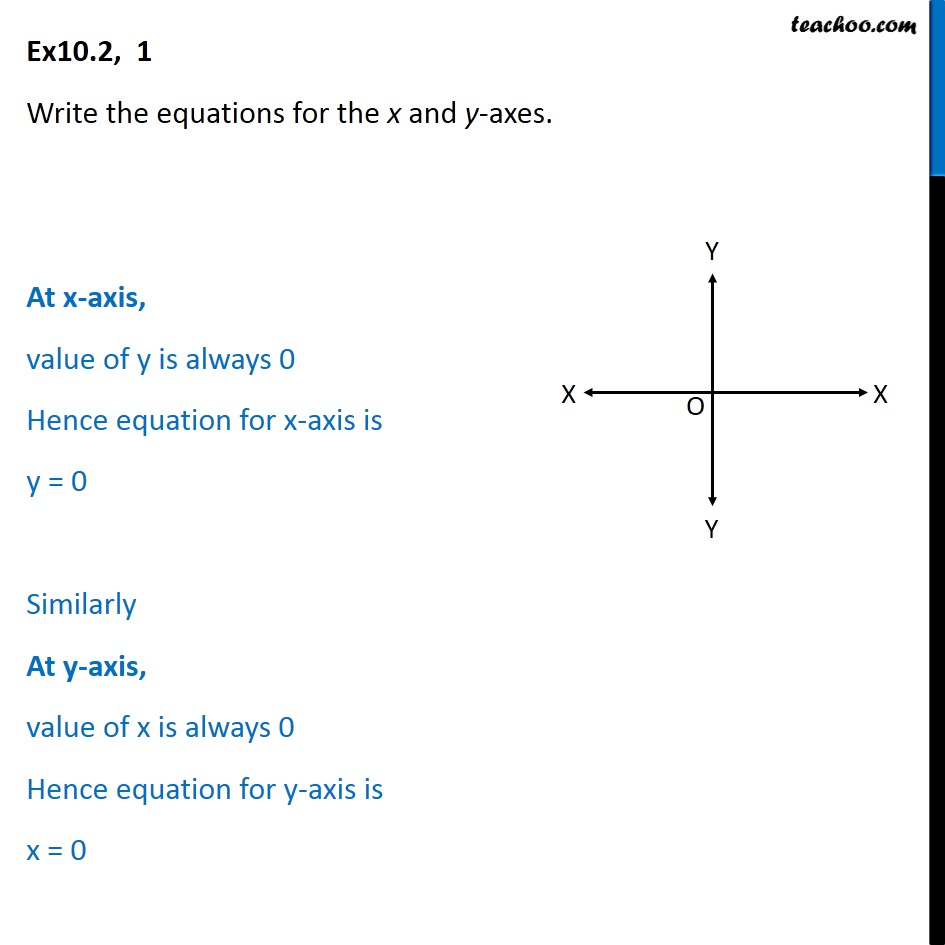Ex 9.2

Chapter 9 Class 11 Straight Lines
Serial order wiseLearn in your speed, with individual attention - Teachoo Maths 1-on-1 Class

### Transcript

Ex10.2, 1 Write the equations for the x and y-axes. At x-axis, value of y is always 0 Hence equation for x-axis is y = 0 Similarly At y-axis, value of x is always 0 Hence equation for y-axis is x = 0